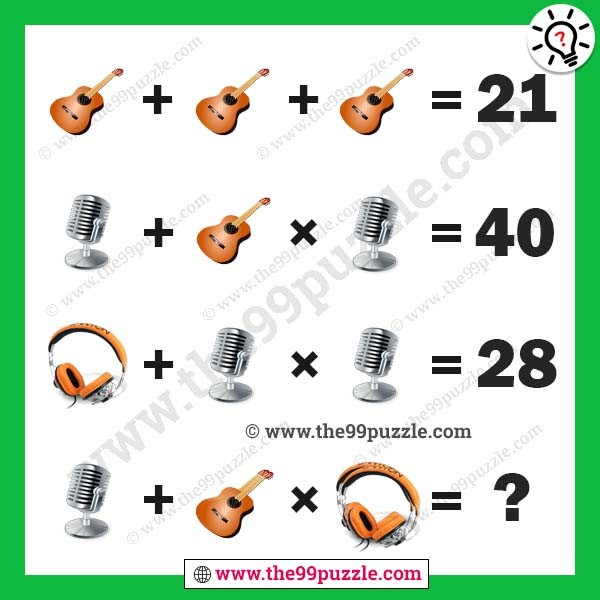# Music picture math equation with answer – Puzz176

Music picture math equation with answer. Can you solve these maths equations to find the value of each of these music instruments and then solve the last question to find the number which will replace the question mark?###### Explanation:

Guiter + Guiter + Guiter = 21

Microphone + Guiter × Microphone = 40

Headphone + Microphone × Microphone = 28

Microphone + Guiter × Microphone = ?

7+7+7 = 21

5+7×5 = 40

3+5×5 = 28

5+(7+7)×(3+3) = 89